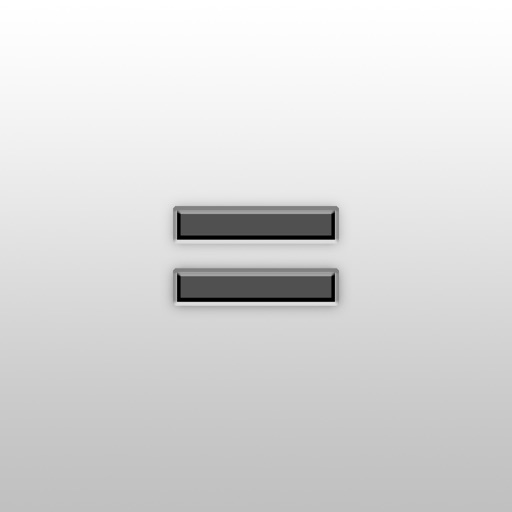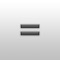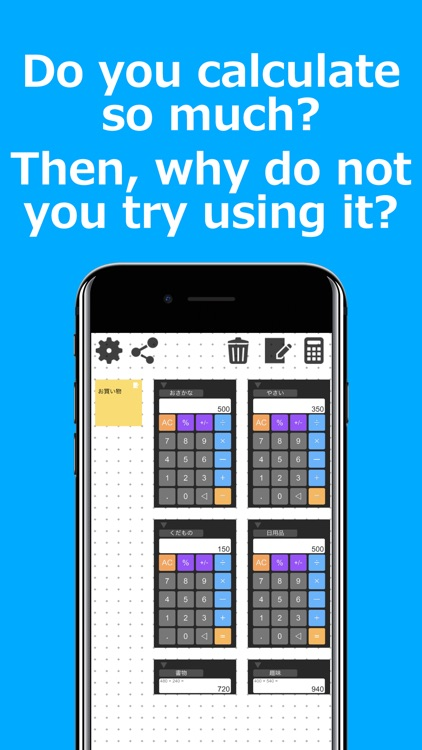## It is a new sense calculator that can arrange calculators and memos with basic functions as much as you want# Calc Table

by Kenjirou UesakaIt is a new sense calculator that can arrange calculators and memos with basic functions as much as you want.### App Details

Version
1.0.2
Rating
NA
Size
41Mb
Genre
Utilities Productivity
Last updated
October 19, 2017
Release date
October 17, 2017

### App Store Description

It is a new sense calculator that can arrange calculators and memos with basic functions as much as you want.
Of course you can use all functions free of charge.

Create a calculator, give it a name, move freely over the field,
It also has a function to compute selected calculators collectively.

It is perfect for you to calculate simple calculator calculator app.

· Basic functions of the calculator
Arithmetic operation (addition, subtraction, multiplication, division)
Percentage calculation
Decimal calculation
Back key that can be traced back to the formula
By pressing equals multiple times at the time of calculation, the previous calculation is performed

· Useful functions
Placing notes
Move calculator or memo
Select range to select calculator and memo at the same time
Calculate the selected calculator at once (long press the calculator addition button)
Calculate calculator and calculator
▼ Compact Calculator Size with Button
Even if you close the application, the calculation result remains intact

Disclaimer:
AppAdvice does not own this application and only provides images and links contained in the iTunes Search API, to help our users find the best apps to download. If you are the developer of this app and would like your information removed, please send a request to [email protected] and your information will be removed.